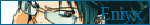Energy and Momentum Problem Link | byon 2011-01-25 21:40:10 (edited 2011-01-25 21:40:50) Hi, I'm in grade 12 physics and i have this momentum and energy problem which i can't solve. I keep encountering imaginary numbers as the answers, please help me. The problem is: A 4kg cart has a spring with a spring constant of 1200 N/m attached to the front. This 4.0kg cart is traveling to the right at 8.0 m/s when it collides in an elastic collision with a 6.0kg cart traveling at 2.0 m/s to the right before the collision. calculate the speed of each cart after the collision and the velocity of each cart when the spring is compressed by the maximum amount also find the maximum amount that the spring is compressed during this collision thanks in advance EnIyX

 Re: Energy and Momentum Problem Link | by Özil on 2011-03-09 22:16:38 try this . 1/2 * K * x^2 = KE(initial)-KE(final) ,where KE final=1/2* total mass(4+10)* [Velocity of centre of mass(44/10)]^2. hence you will find x. velocity of both blocks when spring is compressed maximum is equal to velocity of cente of mass.

 Re: Energy and Momentum Problem Link | by tommorner on 2017-08-11 13:06:49 that one is wrong, as for me